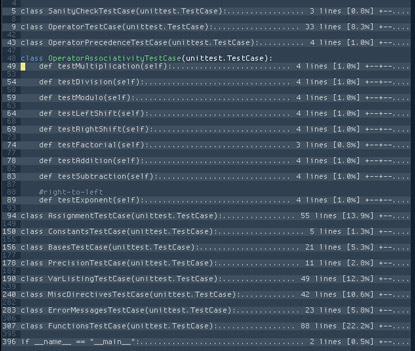I just recently got irritated enough with the current way that folds appear in Vim that I decided to roll my own custom foldtext function.

I wanted the top (non-blank) line to be displayed clearly and cleanly. I also wanted to know not just how many lines were folded but the percentage of the file this accounts for. This is often useful for quickly glancing at to see where most of the document resides. I also wanted a nice easy way of visualising the fold level and how that compares to the folds surrounding a fold.

This is the end result.

```fu! CustomFoldText()
"get first non-blank line
let fs = v:foldstart
while getline(fs) =~ '^\s*\$' | let fs = nextnonblank(fs + 1)
endwhile
if fs > v:foldend
let line = getline(v:foldstart)
else
let line = substitute(getline(fs), '\t', repeat(' ', &tabstop), 'g')
endif

let w = winwidth(0) - &foldcolumn - (&number ? 8 : 0)
let foldSize = 1 + v:foldend - v:foldstart
let foldSizeStr = " " . foldSize . " lines "
let foldLevelStr = repeat("+--", v:foldlevel)
let lineCount = line("\$")
let foldPercentage = printf("[%.1f", (foldSize*1.0)/lineCount*100) . "%] "
let expansionString = repeat(".", w - strwidth(foldSizeStr.line.foldLevelStr.foldPercentage))
return line . expansionString . foldSizeStr . foldPercentage . foldLevelStr
endf
```

And these are the folds that it produces.